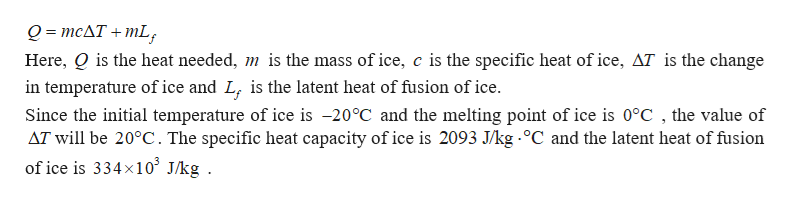# How much heat does it take to completely melt 4 kg of ice, initially at -20oC?

Question
1 views

How much heat does it take to completely melt 4 kg of ice, initially at -20oC?

check_circle

Step 1

The heat needed to melt the ice completely will be the sum of the heat needed to raise the temperature of th...help_outlineImage TranscriptioncloseО - тсдT + тL, Here, Q is the heat needed, m is the mass of ice, c is the specific heat of ice, AT is the change in temperature of ice and L, is the latent heat of fusion of ice. Since the initial temperature of ice is -20°C and the melting point of ice is 0°C , the value of AT will be 20°C. The specific heat capacity of ice is 2093 J/kg °C and the latent heat of fusion of ice is 334x10° J/kg . fullscreen

### Want to see the full answer?

See Solution

#### Want to see this answer and more?

Solutions are written by subject experts who are available 24/7. Questions are typically answered within 1 hour.*

See Solution
*Response times may vary by subject and question.
Tagged in

### Physics